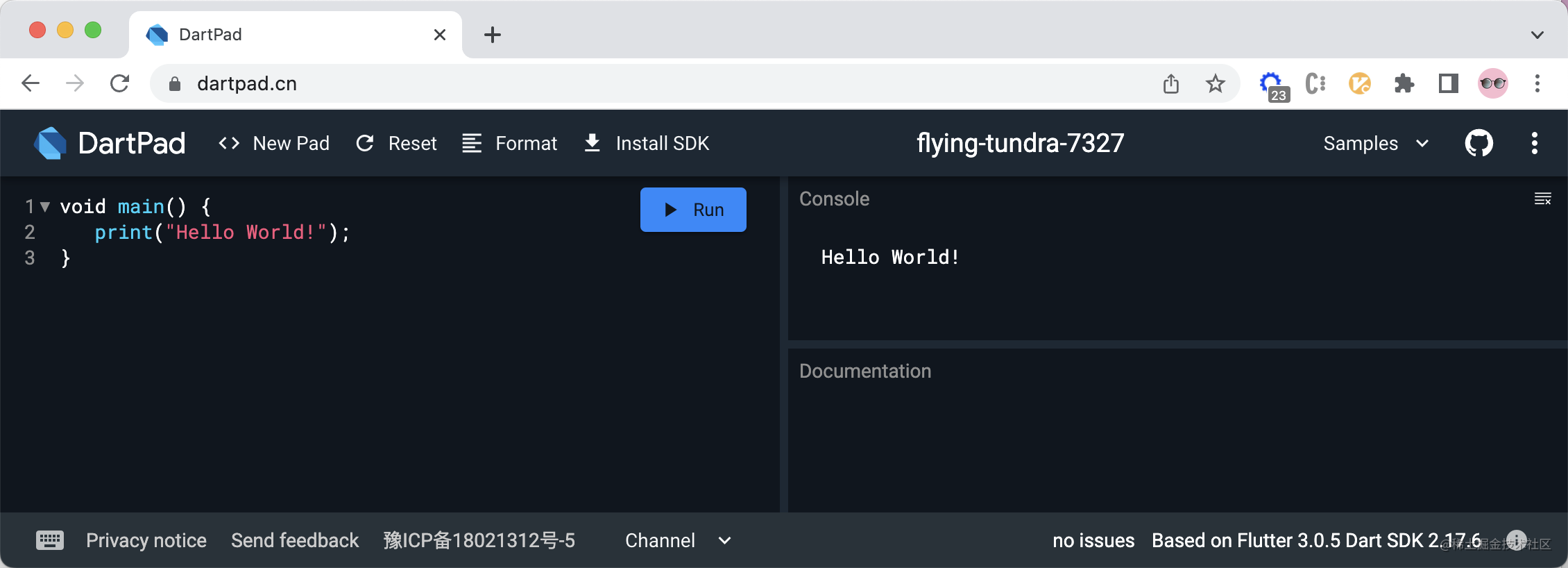# Dart 知識點 - 資料型別

Dart 這個知識點，會寫成一個系列的文章。我們以資料型別開始～### Number 數字型別

• Number 數字型別，包含
• int 整型
• double 浮點型

`int``double` 都是 num 的子類。

```bash int a = 1; print(a); // 1

double b = 2.0; print(b); // 2.0

num c = -3; print(c); // -3 ```

### String 字串型別

`Dart` 支援使用雙引號和單引號來建立字串。使用 `String` 來定義變數，表明該變數是字串型別。`Dart` 中字串型別的使用跟 `JavaScript` 中字串的使用相似：

```bash String s1 = 'Hello '; String s2 = 'World!'; String s3 = s1 + s2; print(s3); // Hello World!```

```bash String s1 = 'I\'m Jimmy.' print(s1); // I'm Jimmy.

String s2 = "第一行文字\n第二行文字"; print(s2); // 第一行文字 // 第二行文字 ```

```bash ''' 第一行文字 第二行文字 ''' // 或者 """ 第一行文字 第二行文字 """```

```bash String s1 = 'my name is Jimmy.'; print('Hello, ' + s1); // Hello, my name is Jimmy. print('Hello, \${ s1 }'); // Hello, my name is Jimmy. print('Hello, \${ s1.substring(10, 1) }!'); // Hello, Jimmy.```

### Boolean 布林型別

```bash String str = ''; if(str) { print('true'); } else { print('flase'); }```

```bash Error: A value of type 'String' can't be assigned to a variable of type 'bool'. if(str) { ^ Error: Compilation failed.````if(str)` 這裡，編譯失敗，因為在 `dart` 語言裡面，變數值必須是 `true` 或者 `false` 才行。改寫下上面的程式碼：`if(str) => if(str.isEmpty)` 即可通過編譯。

| | JavaScript (false) | Dart (true) | | --- | --- | --- | | var variable = 0 | variable （false） | variable <= 0 (true) | | var variable = null | variable （false） | variable == null (true) | | var variable = '' | variable （false） | variable.isEmpty (true) | | var variable = NaN | variable （false） | variable.isNaN (true) | | var variable = true | variable （true） | variable (true) |

```bool flag = true // 或者 bool flag = false```

### list 陣列型別

```bash List arr = ['Hello ', 'World!']; // 陣列的長度 print(arr.length); // 2 // 陣列的取值 print(arr); // Hello // 陣列的賦值 arr = 'Jimmy. '; print(arr.join('')); // Hello Jimmy. // 擴充套件運算子 var list = ["I\'m ", 'come ', 'from ', 'China.']; print([...arr, ...?list].join('')); // Hello Jimmy. I'm come from China.```

`...?` 為空感知擴充套件操作符，如果擴充套件操作符右邊的變數值可能是 `null`， 我們可以使用 `...?` 來避免產生異常

### Set 集合型別

• 無序性：每個元素的地位都是相同的，元素之間無序。
• 互異性：每個元素只能出現一次。
• 確定性：給定一個集合，任何一個元素，該元素`或者屬於或者不屬於`該集合，二者必居其一，不允許模稜兩可的情況出現。

```bash // 定義集合 Set set = new Set(); // 集合的大小 print(set.length); // 0 // 新增元素 set.add('Hello '); set.add('World!'); print(set); // {Hello , World!} // 判斷集合中是否存在指定元素 print(set.contains('Jimmy')); // false // 一次新增多個列表元素 set.addAll(["I\'m ", 'come ', 'from ', 'China.']); print(set); // {Hello , World!, I'm , come , from , China.} // 移除指定的元素 set.remove('World!'); print(set); // {Hello , I'm , come , from , China.}```

### Map 物件型別

`Map` 理解成物件，是因為這跟 `JavaScript` 中的物件很類似。

```bash // 定義物件 Map map = new Map(); // 物件的大小 print(map.length); // 0 // 新增物件屬性 map['name'] = 'Jimmy'; map['country'] = 'China'; print(map); // {name: Jimmy, country: China} // 讀取屬性值 print(map['name']); // Jimmy // 讀取物件的 keys 值 print(map.keys); // (name, country) // 讀取對物件的 values 值 print(map.values); // (Jimmy, China)```

### Runes 和 Symbols

`Dart` 中，runes 公開了字串的 Unicode 碼位。Symbol 表示 Dart 中宣告的操作符或者識別符號。

### 補充

`var``Dart` 語言使用的關鍵字，是 `variable` 的簡寫，表明這是一個泛型呼叫（generic invocations）

```bash var name = 'Jimmy'; // 泛型呼叫，表明變數型別不知道是啥 String name = 'Jimmy'; // 型別註釋，表明我一定是 String 型別```